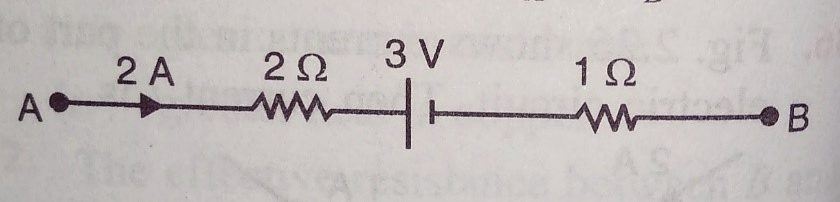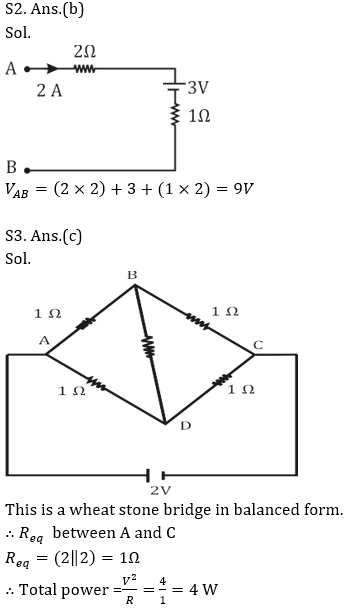Engineering Jobs   »   DFCCIL ELECTRICAL QUIZ

# DFCCIL’21 EE: Daily Practices Quiz 29-July-2021

Each question carries 1 mark.
Negative marking: 1/4 mark
Total Questions: 06
Time: 08 min.

Q1. n similar resistors each of resistance r when connected in parallel have the total
resistance R. When these resistances are connected in series, the total resistance is
(a) nR
(b) R/n²
(c) n²R
(d) R/n

Q2. Fig. shows part of a closed circuit. What is the value of V_A–V_B?(a) 12 V
(b) 9 V
(c) 18 V
(d) 6 V

Q3. A wire of resistance 0.1 Ω /cm is bent to form a square ABCD of side 10 cm. A similar
wire is connected between B and D to form the diagonal BD. If a 2 V battery of negligible
internal resistance is connected between A and C, then total power dissipated is
(a) 2 W
(b) 3 W
(c) 4 W
(d) 6 W

Q4. In a power station,
(a) Reserve capacity = Plant capacity + Max. demand
(b) Reserve capacity = Plant capacity – Average demand
(c) Reserve capacity = Plant capacity – Max. demand
(d) Reserve capacity = Plant capacity + Average demand

Q5. In a 3-phase system, the line losses are
(a) directly proportional to cos ϕ
(b) inversely proportional to cos ϕ
(c) inversely proportional to cos² ϕ
(d) none of the above

Q6. When a 15-V square wave is connected across a 50-V a.c voltmeter, it will read ………
(a) 15 V
(b) 15×√2 V
(c) 15/√2 V
(d) none of the above

SOLUTIONS
S1. Ans.(c)
Sol. In parallel,
R=r/n→r=nR
In series,
R_eq=nr=n²RS4. Ans.(c)
Sol. Reserve capacity = Plant capacity – Max. demand

S5. Ans.(c)
Sol. Line current, I_L=P/(√3 〖 V〗_L cos⁡ϕ )
Since line losses are proportional to the square of I_L, it follows that line losses are proportional to 1/cos² ϕ.

S6. Ans.(a)
Sol. A voltmeter records the r.m.s. value and the r.m.s. value of 15-V square wave is 15 V.

Sharing is caring!

Thank You, Your details have been submitted we will get back to you.